Updating search results...

# 10 Results

View
Selected filters:
• UT.MATH.III.G.MG.2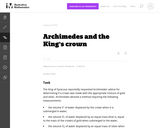Unrestricted Use
CC BY
Rating
0.0 stars

The famous story of Archimedes running through the streets of Syracuse (in Sicily during the third century bc) shouting ''Eureka!!!'' (I have found it) reportedly occurred after he solved this problem. The problem combines the ideas of ratio and proportion within the context of density of matter.

Subject:
Mathematics
Secondary Mathematics
Material Type:
Activity/Lab
Provider:
Illustrative Mathematics
Provider Set:
Illustrative Mathematics
Author:
Illustrative Mathematics
01/04/2013Unrestricted Use
CC BY
Rating
0.0 stars

This is a task from the Illustrative Mathematics website that is one part of a complete illustration of the standard to which it is aligned. Each task has at least one solution and some commentary that addresses important asects of the task and its potential use. Here are the first few lines of the commentary for this task: Eric and Julianne are shoveling snow. After an hour of hard work, Eric remarks ''I bet we have shoveled more than a ton of snow.'' Explain what measure...

Subject:
Mathematics
Secondary Mathematics
Material Type:
Activity/Lab
Provider:
Illustrative Mathematics
Provider Set:
Illustrative Mathematics
Author:
Illustrative Mathematics
01/07/2014Unrestricted Use
CC BY
Rating
0.0 stars

This is a task from the Illustrative Mathematics website that is one part of a complete illustration of the standard to which it is aligned. Each task has at least one solution and some commentary that addresses important asects of the task and its potential use. Here are the first few lines of the commentary for this task: The following clip shows the famous opening scene of the movie Raiders of the Lost Arc. At the beginning of the clip, Indiana Jones is replacing the go...

Subject:
Mathematics
Secondary Mathematics
Material Type:
Activity/Lab
Provider:
Illustrative Mathematics
Provider Set:
Illustrative Mathematics
Author:
Illustrative Mathematics
09/11/2013Unrestricted Use
CC BY
Rating
0.0 stars

The purpose of this task is for students to apply the concepts of mass, volume, and density in a real-world context. There are several ways one might approach the problem, e.g., by estimating the volume of a person and dividing by the volume of a cell.

Subject:
Mathematics
Secondary Mathematics
Material Type:
Activity/Lab
Provider:
Illustrative Mathematics
Provider Set:
Illustrative Mathematics
Author:
Illustrative Mathematics
11/13/2012Unrestricted Use
CC BY
Rating
0.0 stars

This is a mathematical modeling task aimed at making a reasonable estimate for something which is too large to count accurately, the number of leaves on a tree.

Subject:
Mathematics
Secondary Mathematics
Material Type:
Activity/Lab
Provider:
Illustrative Mathematics
Provider Set:
Illustrative Mathematics
Author:
Illustrative Mathematics
01/20/2013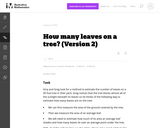Unrestricted Use
CC BY
Rating
0.0 stars

In this problem, the variables a,b,c, and d are introduced to represent important quantities for this esimate: students should all understand where the formula in the solution for the number of leaves comes from. Estimating the values of these variables is much trickier and the teacher should expect and allow a wide range of variation here.

Subject:
Mathematics
Secondary Mathematics
Material Type:
Activity/Lab
Provider:
Illustrative Mathematics
Provider Set:
Illustrative Mathematics
Author:
Illustrative Mathematics
01/20/2013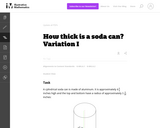Unrestricted Use
CC BY
Rating
0.0 stars

As written, this problem gives students all of the information they need to estimate the thickness of a soda can.

Subject:
Mathematics
Secondary Mathematics
Material Type:
Activity/Lab
Provider:
Illustrative Mathematics
Provider Set:
Illustrative Mathematics
Author:
Illustrative Mathematics
01/04/2013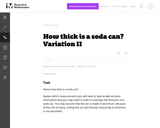Unrestricted Use
CC BY
Rating
0.0 stars

his is a version of ''How thick is a soda can I'' which allows students to work independently and think about how they can determine how thick a soda can is. The teacher should explain clearly that the goal of this task is to come up with an ''indirect'' means of assessing how thick the can is, that is directly measuring its thickness is not allowed.

Subject:
Mathematics
Secondary Mathematics
Material Type:
Activity/Lab
Provider:
Illustrative Mathematics
Provider Set:
Illustrative Mathematics
Author:
Illustrative Mathematics
03/04/2013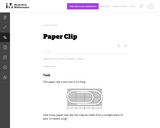Unrestricted Use
CC BY
Rating
0.0 stars

This high level task is an example of applying geometric methods to solve design problems and satisfy physical constraints. This task is accessible to all students. In this task, a typographic grid system serves as the background for a standard paper clip.

Subject:
Mathematics
Secondary Mathematics
Material Type:
Activity/Lab
Provider:
Illustrative Mathematics
Provider Set:
Illustrative Mathematics
Author:
Illustrative Mathematics
05/19/2013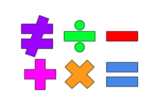Unrestricted Use
Public Domain
Rating
0.0 stars

This lesson is for a math classroom, but can be adapted to fit any grade, subject, or content.&nbsp;&nbsp;In this lesson, students will use an iPad and its features: Keynote, Pages, Garageband, Numbers, Presentation, and iMovie.&nbsp; Students will use 3 of those features/programs to create a video lesson consisting of several examples from a topic of their choice.&nbsp; This project is in place of a term final, so their chosen topic should be from their current term.Image citation: The image is one I created.&nbsp;&nbsp;

Subject:
Secondary Mathematics
Material Type:
Assessment
Author:
Tyrani Bevell